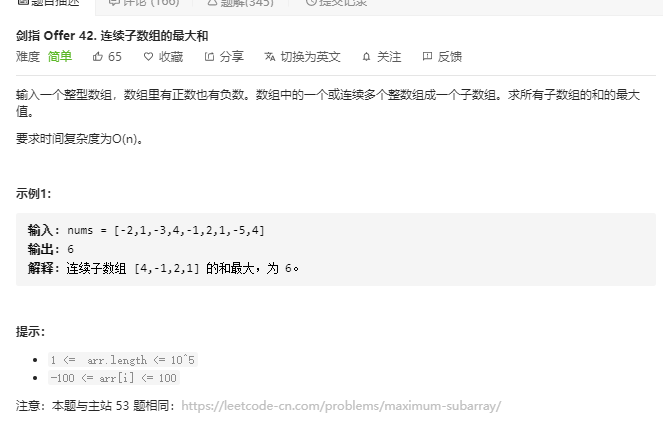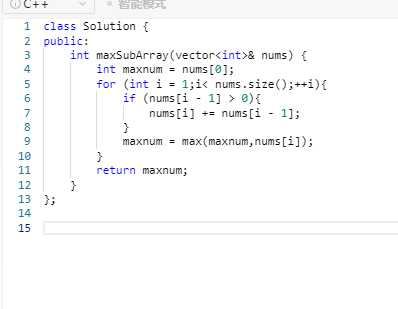## 贪心算法 动态规划/*
* 方法一 贪心法 O(n)
*
* 当叠加的和小于0时，就从下一个数重新开始，
* 同时更新最大和的值(最大值可能为其中某个值)，
* 当叠加和大于0时，将下一个数值加入和中，
* 同时更新最大和的值，依此继续。
*
* 举例： nums = [-2,1,-3,4,-1,2,1,-5,4]
* sum = INT_MIN <= 0-> sum = -2 <= 0 -> sum = 1 > 0 ->
* -> sum = -2 <= 0 -> sum = 4 > 0 -> sum = 3 > 0 ->
* -> sum = 5 > 0 -> sum = 6 > 0 -> sum = 1 > 0 ->
* -> sum = 5 > 0
* res = [-2, 1, 1, 4, 4, 5, 6, 6, 6]
* 最终返回 res = 6
* */动态规划

### 复杂度分析

- 时间复杂度：O(N)